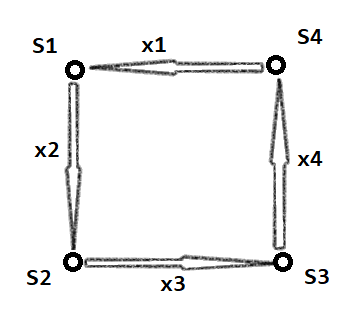# HHUACM 暑假专题 数学

### A - 求递推序列的第N项

$$\left(\begin{matrix} A & B \\ 1 & 0 \\ \end{matrix}\right) \left(\begin{matrix} f(i-1) \\ f(i-2) \\ \end{matrix}\right) =\left(\begin{matrix} f(i) \\ f(i-1) \\ \end{matrix}\right)$$

# 2018广东工业大学校赛题解

### C-平分游戏